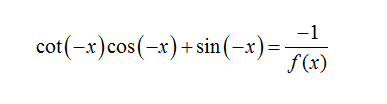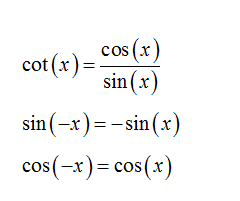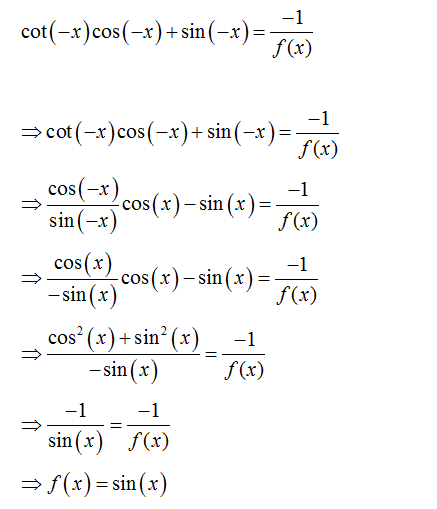# Simplify and write the trigonometric expression in terms of sine and cosine:cot(−x)cos(−x)+sin(−x)=−1/f(x)

Question
22 views

Simplify and write the trigonometric expression in terms of sine and cosine:
cot(−x)cos(−x)+sin(−x)=−1/f(x)

check_circle

Step 1

Given expression is:Step 2

Using trigonometric identities:Step 3

Therefore,...

### Want to see the full answer?

See Solution

#### Want to see this answer and more?

Solutions are written by subject experts who are available 24/7. Questions are typically answered within 1 hour.*

See Solution
*Response times may vary by subject and question.
Tagged in

### Calculus